# Image Deconvolution using Variational Method¶

This numerical tour explores the use of Sobolev and TV regularization to perform image deconvolution.

Important: Please read the installation page for details about how to install the toolboxes. $\newcommand{\dotp}{\langle #1, #2 \rangle}$ $\newcommand{\enscond}{\lbrace #1, #2 \rbrace}$ $\newcommand{\pd}{ \frac{ \partial #1}{\partial #2} }$ $\newcommand{\umin}{\underset{#1}{\min}\;}$ $\newcommand{\umax}{\underset{#1}{\max}\;}$ $\newcommand{\umin}{\underset{#1}{\min}\;}$ $\newcommand{\uargmin}{\underset{#1}{argmin}\;}$ $\newcommand{\norm}{\|#1\|}$ $\newcommand{\abs}{\left|#1\right|}$ $\newcommand{\choice}{ \left\{ \begin{array}{l} #1 \end{array} \right. }$ $\newcommand{\pa}{\left(#1\right)}$ $\newcommand{\diag}{{diag}\left( #1 \right)}$ $\newcommand{\qandq}{\quad\text{and}\quad}$ $\newcommand{\qwhereq}{\quad\text{where}\quad}$ $\newcommand{\qifq}{ \quad \text{if} \quad }$ $\newcommand{\qarrq}{ \quad \Longrightarrow \quad }$ $\newcommand{\ZZ}{\mathbb{Z}}$ $\newcommand{\CC}{\mathbb{C}}$ $\newcommand{\RR}{\mathbb{R}}$ $\newcommand{\EE}{\mathbb{E}}$ $\newcommand{\Zz}{\mathcal{Z}}$ $\newcommand{\Ww}{\mathcal{W}}$ $\newcommand{\Vv}{\mathcal{V}}$ $\newcommand{\Nn}{\mathcal{N}}$ $\newcommand{\NN}{\mathcal{N}}$ $\newcommand{\Hh}{\mathcal{H}}$ $\newcommand{\Bb}{\mathcal{B}}$ $\newcommand{\Ee}{\mathcal{E}}$ $\newcommand{\Cc}{\mathcal{C}}$ $\newcommand{\Gg}{\mathcal{G}}$ $\newcommand{\Ss}{\mathcal{S}}$ $\newcommand{\Pp}{\mathcal{P}}$ $\newcommand{\Ff}{\mathcal{F}}$ $\newcommand{\Xx}{\mathcal{X}}$ $\newcommand{\Mm}{\mathcal{M}}$ $\newcommand{\Ii}{\mathcal{I}}$ $\newcommand{\Dd}{\mathcal{D}}$ $\newcommand{\Ll}{\mathcal{L}}$ $\newcommand{\Tt}{\mathcal{T}}$ $\newcommand{\si}{\sigma}$ $\newcommand{\al}{\alpha}$ $\newcommand{\la}{\lambda}$ $\newcommand{\ga}{\gamma}$ $\newcommand{\Ga}{\Gamma}$ $\newcommand{\La}{\Lambda}$ $\newcommand{\si}{\sigma}$ $\newcommand{\Si}{\Sigma}$ $\newcommand{\be}{\beta}$ $\newcommand{\de}{\delta}$ $\newcommand{\De}{\Delta}$ $\newcommand{\phi}{\varphi}$ $\newcommand{\th}{\theta}$ $\newcommand{\om}{\omega}$ $\newcommand{\Om}{\Omega}$

In :
using PyPlot
using NtToolBox
# arequire("NtToolBox")


This tour is concerned with the deconvolution problem. The measurement are assumed to be blurry and noisy: $$y=\Phi f_0 + w = h \star f_0 + w$$

Where here |h| is the filter (low pass) and |w| some noise (here assumed to be white Gaussian).

We consider variational deconvolution methods, that finds a regularizer through a convex optimization: $$f^\star \in \text{argmin}_f \frac{1}{2}\|y-\Phi f\|^2 + \lambda J(f)$$

Where $J(f)$ is a prior energy. In this tour we consider a simple L2 prior (the image is assumed to have a bounded energy), a Sobolev prior (the image is uniformly smooth) and an approximate total variation (the image has edges of bounded perimeter).

Note that the parameter $\lambda$ should be carefully chosen to fit the noise level.

## Image Blurring¶

Deconvolution corresponds to removing a blur from an image. We use here a Gaussian blur.

First we load the image to be inpainted.

In :
n = 256
name = "NtToolBox/src/data/hibiscus.png"


Initial image $f_0$.

In :
imageplot(f0, "Image f0", [1, 2, 1])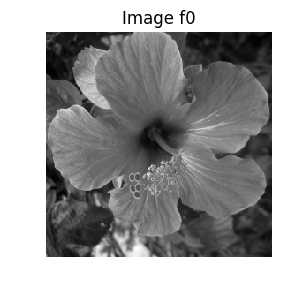Out:
PyObject <matplotlib.text.Text object at 0x326743a50>

We build a convolution kernel. Since we are going to use Fourier to compute the convolution, we set the center of the kernel in the (1,1) pixel location.

Width $s$ of the kernel, in pixel.

In :
s = 3;


Define the convolution kernel $h$.

In :
include("NtToolBox/src/ndgrid.jl")
x = [collect(0:n/2 - 1); collect(-n/2:-1)]
(Y, X) = meshgrid(x, x)
h = exp((-X.^2 - Y.^2)/(2*s^2))
h = h/sum(h);


Useful for later : the Fourier transform (should be real because of symmetry).

In :
hF = real(plan_fft(h)*h);

Out:
256×256 Array{Float64,2}:
1.0       0.997293  0.989216  0.975898  …  0.975898  0.989216  0.997293
0.997293  0.994593  0.986538  0.973256     0.973256  0.986538  0.994593
0.989216  0.986538  0.978547  0.965374     0.965374  0.978547  0.986538
0.975898  0.973256  0.965374  0.952377     0.952377  0.965374  0.973256
0.957555  0.954963  0.947228  0.934476     0.934476  0.947228  0.954963
0.934476  0.931946  0.924398  0.911954  …  0.911954  0.924398  0.931946
0.907023  0.904567  0.897241  0.885162     0.885162  0.897241  0.904567
0.875616  0.873246  0.866173  0.854512     0.854512  0.866173  0.873246
0.840726  0.83845   0.831659  0.820463     0.820463  0.831659  0.83845
0.802862  0.800689  0.794204  0.783512     0.783512  0.794204  0.800689
0.762558  0.760494  0.754334  0.744179  …  0.744179  0.754334  0.760494
0.720361  0.718411  0.712592  0.702999     0.702999  0.712592  0.718411
0.67682   0.674987  0.66952   0.660507     0.660507  0.66952   0.674987
⋮                                       ⋱                      ⋮
0.67682   0.674987  0.66952   0.660507     0.660507  0.66952   0.674987
0.720361  0.718411  0.712592  0.702999  …  0.702999  0.712592  0.718411
0.762558  0.760494  0.754334  0.744179     0.744179  0.754334  0.760494
0.802862  0.800689  0.794204  0.783512     0.783512  0.794204  0.800689
0.840726  0.83845   0.831659  0.820463     0.820463  0.831659  0.83845
0.875616  0.873246  0.866173  0.854512     0.854512  0.866173  0.873246
0.907023  0.904567  0.897241  0.885162  …  0.885162  0.897241  0.904567
0.934476  0.931946  0.924398  0.911954     0.911954  0.924398  0.931946
0.957555  0.954963  0.947228  0.934476     0.934476  0.947228  0.954963
0.975898  0.973256  0.965374  0.952377     0.952377  0.965374  0.973256
0.989216  0.986538  0.978547  0.965374     0.965374  0.978547  0.986538
0.997293  0.994593  0.986538  0.973256  …  0.973256  0.986538  0.994593

Display the kernel and its transform. We use |fftshift| to center the filter for display.

In :
imageplot(fftshift(h), "Filter", [1, 2, 1])
imageplot(fftshift(hF), "Fourier transform", [1, 2, 2])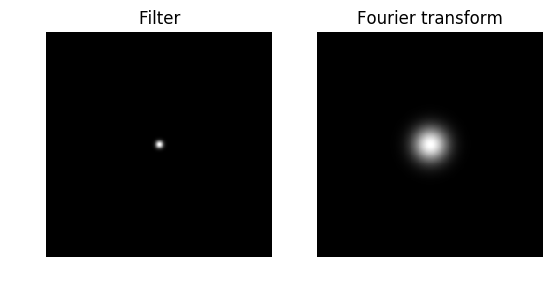Out:
PyObject <matplotlib.text.Text object at 0x000000001DAD18D0>

We use this short hand for the filtering. Note that this is a symmetric operator.

In :
Phi = (x, h) -> real(plan_ifft((plan_fft(x)*x) .* (plan_fft(h)*h))*((plan_fft(x)*x) .* (plan_fft(h)*h)))

Out:
(::#3) (generic function with 1 method)

Important Scilab user should define a function |Phi| in a separate file |Phi.sci| to perform this.

Apply the filter.

In :
y0 = Phi(f0, h);

Out:
256×256 Array{Float64,2}:
0.147006  0.164445  0.178005  0.187653  …  0.0906591  0.107472   0.127165
0.138294  0.156249  0.170627  0.181218     0.0822509  0.0987673  0.118306
0.132873  0.151598  0.166987  0.178637     0.0760766  0.0926947  0.112434
0.131464  0.151143  0.167608  0.180264     0.0728161  0.0899615  0.110277
0.133701  0.154393  0.171868  0.185341     0.0725241  0.0904672  0.111599
0.138475  0.160082  0.17837   0.192364  …  0.0747425  0.0935097  0.11548
0.144456  0.166724  0.185502  0.199624     0.0787502  0.098146   0.12077
0.150461  0.172999  0.191824  0.205601     0.0837858  0.103476   0.126429
0.155538  0.177844  0.196176  0.20908      0.0891548  0.108739   0.131609
0.158878  0.180365  0.197607  0.209119     0.0942428  0.113281   0.135589
0.159786  0.179831  0.195408  0.205105  …  0.0985227  0.116535   0.137736
0.157835  0.17587   0.189329  0.196972     0.101614   0.118099   0.137626
0.153118  0.168747  0.179862  0.185461     0.103376   0.117879   0.135232
⋮                                       ⋱                        ⋮
0.211392  0.227374  0.239281  0.245411     0.163151   0.176982   0.193746
0.208349  0.225184  0.238373  0.24617   …  0.159525   0.173321   0.190243
0.204261  0.221724  0.235947  0.245225     0.155038   0.168817   0.185842
0.19968   0.21752   0.232441  0.242862     0.150085   0.163933   0.181068
0.195207  0.213193  0.228429  0.239543     0.145006   0.159105   0.17645
0.191305  0.209261  0.224435  0.235735     0.140027   0.154636   0.172375
0.188057  0.205868  0.220664  0.231677  …  0.135161   0.150538   0.168877
0.184983  0.202593  0.216809  0.227195     0.13015    0.146431   0.165494
0.181112  0.198512  0.212106  0.221746     0.124497   0.141595   0.161332
0.175394  0.192616  0.20572   0.214754     0.117678   0.135268   0.155434
0.167307  0.184435  0.197327  0.206113     0.109455   0.127084   0.147325
0.157342  0.17452   0.187562  0.196563  …  0.100144   0.117432   0.137446

Display the filtered observation.

In :
imageplot(y0, "Observation without noise", [1, 2, 2])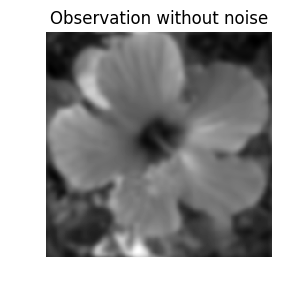Out:
PyObject <matplotlib.text.Text object at 0x000000001EB40CC0>

Variance $\sigma^2$ of the noise $w$.

In :
sigma = .02;

Out:
0.02

Add some noise to obtain the measurements $y = \Phi f_0 + w$.

In :
y = y0 .+ randn(n, n).*sigma;

Out:
256×256 Array{Float64,2}:
0.187654   0.185974  0.195066  0.189263  …  0.10219    0.0918291  0.116488
0.0948482  0.164217  0.154785  0.205916     0.114538   0.0904093  0.121988
0.109873   0.151707  0.152401  0.174968     0.0855326  0.107687   0.123125
0.143554   0.127152  0.145506  0.202726     0.0730925  0.0606067  0.11371
0.140267   0.162569  0.12932   0.216793     0.0631901  0.0337215  0.0986603
0.115588   0.177814  0.179761  0.207952  …  0.0853762  0.116003   0.112846
0.109074   0.185258  0.207259  0.168357     0.0715336  0.101621   0.128139
0.1629     0.186155  0.178142  0.204365     0.0927259  0.11247    0.159682
0.188056   0.179991  0.196923  0.204523     0.0847032  0.0837348  0.142779
0.152072   0.189626  0.251811  0.183494     0.0361014  0.0995018  0.162877
0.161646   0.158808  0.189759  0.200411  …  0.0688726  0.104941   0.135166
0.18464    0.179556  0.19524   0.191587     0.100401   0.145377   0.141307
0.149682   0.151738  0.186778  0.19889      0.104088   0.128602   0.0990785
⋮                                        ⋱                        ⋮
0.211564   0.223764  0.225694  0.244671     0.149713   0.189644   0.230029
0.195579   0.255084  0.241725  0.275118  …  0.189193   0.185328   0.175083
0.222678   0.216384  0.21176   0.229708     0.154533   0.188109   0.180246
0.180241   0.184694  0.247267  0.238275     0.169057   0.149369   0.146512
0.173484   0.216062  0.249936  0.180448     0.113218   0.144172   0.18361
0.185531   0.247864  0.19006   0.262168     0.115784   0.145987   0.237863
0.212075   0.212495  0.226593  0.254123  …  0.149225   0.124584   0.183222
0.189201   0.224105  0.184032  0.276107     0.115665   0.154473   0.132736
0.198049   0.181465  0.18256   0.20032      0.131819   0.128139   0.162517
0.193973   0.181978  0.203586  0.187119     0.103915   0.129151   0.153031
0.137058   0.205306  0.190603  0.177478     0.106389   0.17353    0.177976
0.165238   0.177609  0.206904  0.214931  …  0.0620544  0.117736   0.160573 

Display.

In :
imageplot(clamP(y), "Observation with noise", [1, 2, 2])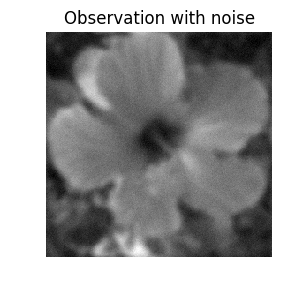Out:
PyObject <matplotlib.text.Text object at 0x000000001ED8D2E8>
In :
imageplot(y, string("Observation, SNR = ", string(round(snr(f0, y),2)), "dB"))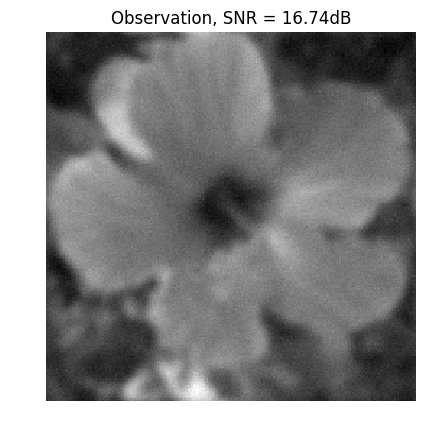Out:
PyObject <matplotlib.text.Text object at 0x000000001EFE0EF0>

## Deconvolution with L2 Regularization¶

Deconvolution is obtained by dividing the Fourier transform of $y$ by $\hat h$. $$f^\star(\omega) = \frac{\hat y(\omega)}{\hat h(\omega)} = \hat f_0(\omega) + \hat w(\omega)/{\hat h(\omega)}$$

Unfortunately this creates an explosion of the Noise.

To avoid this explosion, we consider a simple regularization. $$f^{\star} = \text{argmin}_f \: \|y-\Phi f\|^2 + \lambda \|f\|^2$$

Since the filtering is diagonalized over Fourier, the solution is simply computed over the Fourier domain as: $$\hat f^\star(\omega) = \frac{\hat y(\omega) \hat h(\omega)}{ \|\hat h(\omega)\|^2 + \lambda }$$

Useful for later: Fourier transform of the observations.

In :
yF = plan_fft(y)*y;

Out:
256×256 Array{Complex{Float64},2}:
23959.6+0.0im         -2143.98-880.931im  …     -2143.98+880.931im
-2229.31-216.21im      -2522.91-1518.67im        -1420.11+682.078im
-997.392-22.7833im     -203.708+500.58im          426.197+1008.44im
327.986+392.848im     -119.196-345.563im        -222.064-48.2954im
-84.1553+594.889im      54.5129+162.707im         348.778+164.008im
341.862+194.015im      254.462-18.3943im  …      122.646-71.5433im
-118.367+375.152im      114.547+158.468im        -319.528+64.7372im
-96.1416+209.634im      296.944+220.954im        -314.014+55.2186im
95.7686+199.581im      33.0256+1.18373im         2.73406+86.182im
-25.4992+154.863im      75.9527-159.491im        -105.829+76.8562im
-103.789+201.114im  -0.00158153+79.6004im  …     -78.2486+4.80056im
-140.109+49.5548im      -81.907-23.7371im        -14.7995-4.43073im
102.193+2.29866im      64.3186-7.02616im         19.1352-125.278im
⋮                                  ⋱             ⋮
102.193-2.29866im      19.1352+125.278im         64.3186+7.02616im
-140.109-49.5548im     -14.7995+4.43073im  …      -81.907+23.7371im
-103.789-201.114im     -78.2486-4.80056im     -0.00158153-79.6004im
-25.4992-154.863im     -105.829-76.8562im         75.9527+159.491im
95.7686-199.581im      2.73406-86.182im          33.0256-1.18373im
-96.1416-209.634im     -314.014-55.2186im         296.944-220.954im
-118.367-375.152im     -319.528-64.7372im  …      114.547-158.468im
341.862-194.015im      122.646+71.5433im         254.462+18.3943im
-84.1553-594.889im      348.778-164.008im         54.5129-162.707im
327.986-392.848im     -222.064+48.2954im        -119.196+345.563im
-997.392+22.7833im      426.197-1008.44im        -203.708-500.58im
-2229.31+216.21im      -1420.11-682.078im  …     -2522.91+1518.67im

Select a value for the regularization parameter.

In :
Lambda = 0.02;

Out:
0.02

Perform the inversion.

In :
fL2 = real(plan_ifft((yF .* hF ./ (abs(hF).^2 .+ Lambda)))*((yF .* hF ./ (abs(hF).^2 .+ Lambda))));

Out:
256×256 Array{Float64,2}:
0.162544  0.179298  0.184509  0.182516  …  0.0698412   0.101146   0.134711
0.135278  0.157158  0.169893  0.175841     0.0451367   0.0734671  0.105721
0.113034  0.140381  0.160654  0.174409     0.0224082   0.0489482  0.0809874
0.10342   0.135526  0.161482  0.180579     0.00818441  0.0348732  0.0682827
0.109804  0.145305  0.174012  0.194632     0.00625252  0.034968   0.0712569
0.129587  0.167085  0.195777  0.214339  …  0.0157824   0.0475732  0.0876302
0.155517  0.194114  0.221244  0.235814     0.0319167   0.066651   0.110449
0.179103  0.218471  0.244     0.254771     0.0485613   0.085039   0.131588
0.194054  0.233982  0.258777  0.267442     0.0615229   0.0978598  0.145318
0.197972  0.237758  0.262571  0.271061     0.0699496   0.104023   0.149942
0.191946  0.230142  0.254865  0.264238  …  0.0754737   0.105323   0.147066
0.178988  0.213717  0.237333  0.247373     0.0802253   0.104373   0.139668
0.162592  0.192233  0.213362  0.222933     0.0853638   0.103043   0.130509
⋮                                       ⋱                         ⋮
0.2269    0.255543  0.27548   0.283871     0.145187    0.165838   0.194862
0.213799  0.242036  0.263361  0.275427  …  0.135923    0.155495   0.183087
0.200189  0.228668  0.251672  0.267416     0.123613    0.142771   0.169884
0.191051  0.220268  0.244587  0.262905     0.111601    0.131761   0.159941
0.18877   0.218337  0.242488  0.261082     0.102819    0.125593   0.156202
0.192417  0.220905  0.2425    0.258419     0.0986966   0.1252     0.158762
0.199078  0.224571  0.241139  0.2517    …  0.0990011   0.129457   0.165528
0.20576   0.226795  0.236785  0.240448     0.102249    0.136053   0.173613
0.210154  0.226498  0.229984  0.22685      0.106059    0.142104   0.180101
0.210058  0.222921  0.221744  0.213623     0.107349    0.144282   0.181917
0.202902  0.214553  0.21191   0.202005     0.102847    0.139183   0.17586
0.186768  0.199831  0.199463  0.191699  …  0.0902727   0.124573   0.159808 

Display.

In :
imageplot(clamP(fL2), string("L2 deconvolution, SNR = ", string(round(snr(f0, fL2), 2)), "dB"))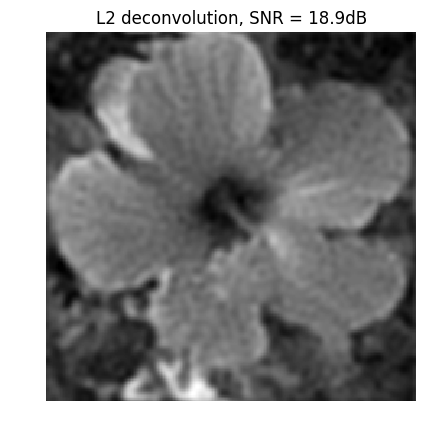Out:
PyObject <matplotlib.text.Text object at 0x0000000026C12400>

Exercise 1: Find the optimal solution fL2 by testing several value of $\lambda$.

In :
include("NtSolutions/inverse_2_deconvolution_variational/exo1.jl")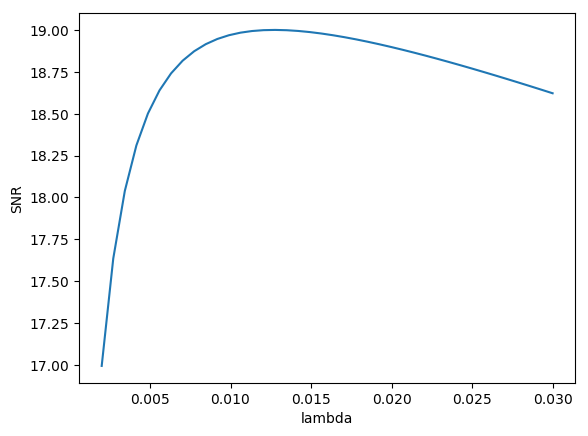Out:
256×256 Array{Float64,2}:
0.172828   0.188661  0.189746  …   0.0698061    0.104854   0.142608
0.139417   0.161483  0.172343      0.0424529    0.0728089  0.107817
0.11129    0.13975   0.160133      0.0165905    0.0438522  0.077498
0.0987255  0.132439  0.159574      0.000476029  0.0274102  0.0619509
0.106348   0.143443  0.17319      -0.00108197   0.0282633  0.0659985
0.130656   0.169416  0.198056  …   0.0106395    0.0439784  0.0864246
0.161938   0.20154   0.227281      0.0291259    0.0663883  0.113822
0.189174   0.229719  0.253056      0.046588     0.0862457  0.137617
0.204775   0.246547  0.269297      0.0583074    0.0979514  0.151021
0.206657   0.24916   0.272889      0.0643789    0.101348   0.153009
0.197225   0.238765  0.263663  …   0.0680819    0.0999811  0.146821
0.180859   0.218998  0.243811      0.0727651    0.0978384  0.136891
0.161877   0.19444   0.217286      0.0797671    0.097142   0.126597
⋮                              ⋱                           ⋮
0.232417   0.262507  0.282589      0.145385     0.167027   0.198121
0.215994   0.244587  0.265486  …   0.135835     0.155678   0.184272
0.199465   0.227822  0.2503        0.122129     0.141129   0.168733
0.189391   0.218675  0.242689      0.108293     0.128516   0.157556
0.188518   0.218625  0.242563      0.0978814    0.121671   0.154298
0.195036   0.224281  0.245171      0.0926359    0.121604   0.158707
0.204818   0.230691  0.245318  …   0.0924668    0.126931   0.16794
0.214293   0.234799  0.241102      0.0960849    0.135199   0.178675
0.221291   0.236055  0.234107      0.101288     0.143523   0.187957
0.223645   0.234202  0.226406      0.104803     0.148288   0.192479
0.21798    0.227216  0.217736      0.102555     0.145174   0.188104
0.200999   0.212174  0.205861  …   0.0910958    0.1307     0.171386 

Display optimal result.

In :
imageplot(clamP(fL2), string("L2 deconvolution, SNR = ", string(round(snr(f0, fL2), 2)), "dB") )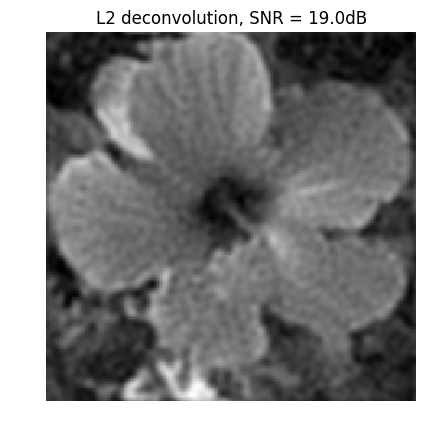Out:
PyObject <matplotlib.text.Text object at 0x0000000026FE78D0>

## Deconvolution by Sobolev Regularization.¶

L2 regularization did not perform any denoising. To remove some noise, we can penalize high frequencies using Sobolev regularization (quadratic grow).

The Sobolev prior reads (note the conversion from spacial domain to Fourier domain) $$J(f) = \sum_x \|\nabla f(x)\|^2 = \sum_{\omega} S(\omega) \|\hat f(\omega)\|^2$$ where $S(\omega)=\|\omega\|^2$.

Since this prior can be written over the Fourier domain, one can compute the solution to the deblurring with Sobolev prior simply with the Fourier coefficients: $$\hat f^\star(\omega) = \frac{\hat y(\omega) \hat h(\omega)}{ \|\hat h(\omega)\|^2 + \lambda S(\omega) }$$

Compute the Sobolev prior penalty |S|(rescale to [0,1]).

In :
S = (X.^2 + Y.^2) .* (2/n).^2;

Out:
256×256 Array{Float64,2}:
0.0          6.10352e-5   0.000244141  …  0.000244141  6.10352e-5
6.10352e-5   0.00012207   0.000305176     0.000305176  0.00012207
0.000244141  0.000305176  0.000488281     0.000488281  0.000305176
0.000549316  0.000610352  0.000793457     0.000793457  0.000610352
0.000976563  0.0010376    0.0012207       0.0012207    0.0010376
0.00152588   0.00158691   0.00177002   …  0.00177002   0.00158691
0.00219727   0.0022583    0.00244141      0.00244141   0.0022583
0.00299072   0.00305176   0.00323486      0.00323486   0.00305176
0.00390625   0.00396729   0.00415039      0.00415039   0.00396729
0.00494385   0.00500488   0.00518799      0.00518799   0.00500488
0.00610352   0.00616455   0.00634766   …  0.00634766   0.00616455
0.00738525   0.00744629   0.00762939      0.00762939   0.00744629
0.00878906   0.0088501    0.0090332       0.0090332    0.0088501
⋮                                      ⋱               ⋮
0.00878906   0.0088501    0.0090332       0.0090332    0.0088501
0.00738525   0.00744629   0.00762939   …  0.00762939   0.00744629
0.00610352   0.00616455   0.00634766      0.00634766   0.00616455
0.00494385   0.00500488   0.00518799      0.00518799   0.00500488
0.00390625   0.00396729   0.00415039      0.00415039   0.00396729
0.00299072   0.00305176   0.00323486      0.00323486   0.00305176
0.00219727   0.0022583    0.00244141   …  0.00244141   0.0022583
0.00152588   0.00158691   0.00177002      0.00177002   0.00158691
0.000976563  0.0010376    0.0012207       0.0012207    0.0010376
0.000549316  0.000610352  0.000793457     0.000793457  0.000610352
0.000244141  0.000305176  0.000488281     0.000488281  0.000305176
6.10352e-5   0.00012207   0.000305176  …  0.000305176  0.00012207 

Regularization parameter:

In :
Lambda = 0.2;

Out:
0.2

Perform the inversion.

In :
fSob = real(plan_ifft((yF .* hF ./ (abs(hF).^2 .+ Lambda.*S)))*((yF .* hF ./ (abs(hF).^2 .+ Lambda.*S))));

Out:
256×256 Array{Float64,2}:
0.175639  0.19019   0.189784  0.181096  …   0.0709781   0.107597   0.145925
0.142296  0.163247  0.172283  0.17375       0.04087     0.0736867  0.110397
0.114817  0.142669  0.161192  0.172556      0.012912    0.0433002  0.0797346
0.102337  0.13616   0.161862  0.180008     -0.00453659  0.025591   0.0634812
0.10899   0.147008  0.176298  0.196541     -0.00673413  0.0252544  0.0661898
0.13184   0.172287  0.201825  0.219858  …   0.00520149  0.040325   0.0852486
0.162261  0.204053  0.232024  0.245588      0.0250944   0.0633587  0.112266
0.189869  0.232701  0.25916   0.268613      0.0451309   0.0853097  0.137162
0.206721  0.25054   0.276711  0.28428       0.0597676   0.0997531  0.152552
0.20969   0.253931  0.280955  0.289184      0.0677859   0.105067   0.156083
0.200318  0.243476  0.271401  0.281705  …   0.071598    0.103755   0.149966
0.182984  0.222846  0.250421  0.262465      0.0747898   0.0999625  0.138687
0.162767  0.197208  0.222495  0.234591      0.0797267   0.0970127  0.126604
⋮                                       ⋱                          ⋮
0.236245  0.268219  0.290054  0.298505      0.145699    0.168011   0.200319
0.219679  0.250613  0.273607  0.286097  …   0.135257    0.15584    0.185903
0.202416  0.23314   0.257653  0.274186      0.120748    0.1405     0.169585
0.1908    0.22204   0.247695  0.266969      0.10618     0.127043   0.157257
0.188176  0.21968   0.244821  0.264105      0.0953185   0.119534   0.152844
0.194027  0.224296  0.246151  0.261842      0.0904253   0.11966    0.157045
0.205166  0.23199   0.247549  0.256226  …   0.0918311   0.126608   0.167777
0.217575  0.239074  0.246289  0.245842      0.0980331   0.137679   0.181401
0.227359  0.24297   0.241721  0.232146      0.105892    0.148847   0.193553
0.23069   0.241595  0.234037  0.217675      0.110969    0.155188   0.199451
0.223934  0.232792  0.22294   0.204079      0.108478    0.151793   0.194537
0.205025  0.215187  0.207892  0.191758  …   0.0950877   0.135609   0.176188 

Display the result.

In :
imageplot(clamP(fSob), string("Sobolev deconvolution, SNR = ", string( round(snr(f0, fSob), 2) ), "dB") )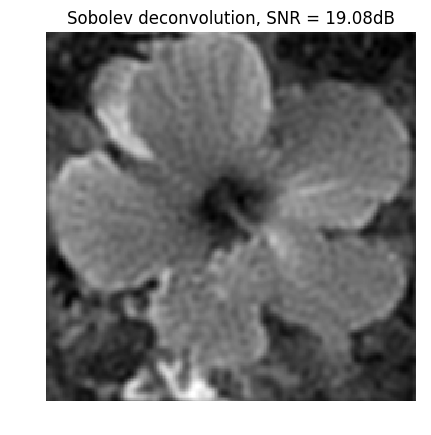Out:
PyObject <matplotlib.text.Text object at 0x0000000026ED0470>

Exercise 2: Find the optimal solution fSob by testing several value of $\lambda$.

In :
include("NtSolutions/inverse_2_deconvolution_variational/exo2.jl")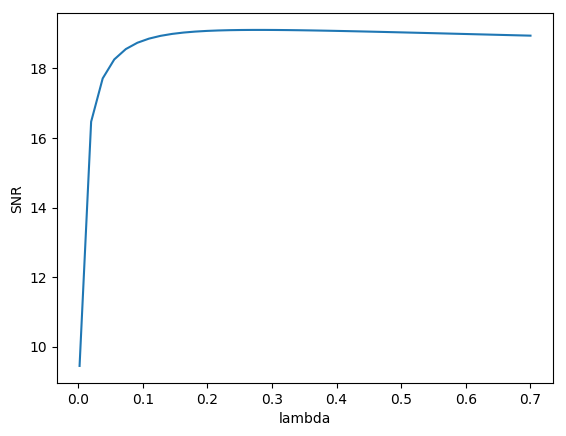Out:
256×256 Array{Float64,2}:
0.16994   0.185339  0.187795  0.182789  …   0.0713346   0.105698   0.141525
0.139974  0.16114   0.171741  0.175358      0.0426857   0.0742002  0.109195
0.115693  0.143219  0.162171  0.174281      0.0164528   0.0462581  0.0815925
0.104836  0.138064  0.1636    0.181721     -2.78932e-5  0.0298136  0.0668898
0.11087   0.148349  0.177575  0.197901     -0.00259494  0.0289657  0.0689966
0.131322  0.171491  0.201608  0.220568  …   0.00791182  0.0421771  0.0857983
0.158885  0.200628  0.229951  0.245543      0.0262935   0.0632087  0.110251
0.18459   0.227347  0.255614  0.267878      0.0457598   0.0842433  0.133678
0.201274  0.244669  0.272532  0.282996      0.0611486   0.0993629  0.149371
0.205678  0.248963  0.277046  0.287538      0.0707465   0.106488   0.154666
0.198563  0.240304  0.268435  0.279956  …   0.0759476   0.107074   0.150807
0.183394  0.221668  0.248715  0.260946      0.0794449   0.104291   0.141283
0.164637  0.197656  0.222     0.233587      0.08333     0.101068   0.129917
⋮                                       ⋱                          ⋮
0.233826  0.265251  0.287269  0.296466      0.145866    0.16768    0.198955
0.219113  0.250198  0.273778  0.286991  …   0.135296    0.15587    0.1856
0.203478  0.234695  0.259935  0.277013      0.121364    0.141406   0.170512
0.192419  0.224082  0.250397  0.270029      0.107817    0.128853   0.15889
0.189074  0.220702  0.246449  0.266123      0.0981163   0.121922   0.154338
0.193324  0.223485  0.246165  0.262677      0.0942646   0.122169   0.157658
0.202561  0.22934   0.246344  0.25671   …   0.096336    0.128765   0.167053
0.213103  0.234948  0.244552  0.24696       0.102556    0.138973   0.179071
0.221128  0.23764   0.239792  0.234272      0.109626    0.148764   0.189445
0.222945  0.235221  0.231811  0.22041       0.113267    0.153467   0.19365
0.215396  0.225801  0.220303  0.206717      0.109265    0.148785   0.187718
0.197073  0.208586  0.205183  0.19388   …   0.095056    0.132413   0.169737 

Display optimal result.

In :
imageplot(clamP(fSob), string("Sobolev deconvolution, SNR = ", string( round(snr(f0, fSob), 2) ), "dB") )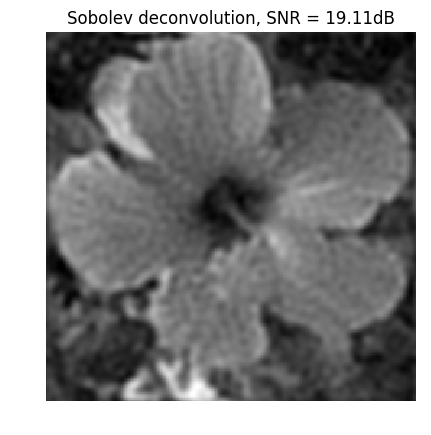Out:
PyObject <matplotlib.text.Text object at 0x0000000027733CC0>

## Deconvolution by Total Variation Regularization¶

Sobolev regularization perform a denoising but also tends to blur the edges, thus producing a poor results on cartoon images.

The TV prior is able to better reconstruct sharp edges. It reads: $$J(f) = \sum_x \| \nabla f(x)\|$$

With respect to the Sobolev energy, it simply corresponding to measuring the L1 norm instead of the L2 norm, thus dropping the square in the functional.

Unfortunately, the TV functional $J(f)$ is not a smooth function of the image $f$. It thus requires the use of advanced convex optimization method to be minimized for regularization.

An alternative is to replace the absolute value by a smooth absolute value. The smoothed TV norm reads: $$J(f) = \sum_x \sqrt{\|\nabla f(x)\|^2+\varepsilon^2}$$

Regularization parameter for the TV norm:

In :
epsilon = 0.4*1e-2;

Out:
0.004

When $\epsilon$ gets close to zero, the smoothed energy becomes closer to the original total variation, but the optimization becomes more difficult. When |epsilon| becomes large, the smoothed energy becomes closer to the Sobolev energy, thus blurring the edges.

Unfortunately, this prior is non-quadratic, and cannot be expressed over the Fourier domain. One thus need to use an iterative scheme such as a gradient descent to approximate the solution.

An iteration of the gradient descent reads: $$f^{(k+1)} = f^{(k)} - \tau \left( h \star (h \star f^{(k)} - y) + \lambda \text{Grad} J(f^{(k)}) \right)$$

Regularization parameter.

In :
Lambda = 0.06;

Out:
0.06

The value of $\tau$, the step size, should be smaller than twice the Lipschitz constant of the Gradient of the functional to be minimized, hence: $$\tau< \frac{2}{1 + \lambda 8/\varepsilon }.$$

In :
tau = 1.9 / (1 + Lambda * 8 / epsilon);

Out:
0.015702479338842973

Initialization.

In :
fTV = y;

Out:
256×256 Array{Float64,2}:
0.187654   0.185974  0.195066  0.189263  …  0.10219    0.0918291  0.116488
0.0948482  0.164217  0.154785  0.205916     0.114538   0.0904093  0.121988
0.109873   0.151707  0.152401  0.174968     0.0855326  0.107687   0.123125
0.143554   0.127152  0.145506  0.202726     0.0730925  0.0606067  0.11371
0.140267   0.162569  0.12932   0.216793     0.0631901  0.0337215  0.0986603
0.115588   0.177814  0.179761  0.207952  …  0.0853762  0.116003   0.112846
0.109074   0.185258  0.207259  0.168357     0.0715336  0.101621   0.128139
0.1629     0.186155  0.178142  0.204365     0.0927259  0.11247    0.159682
0.188056   0.179991  0.196923  0.204523     0.0847032  0.0837348  0.142779
0.152072   0.189626  0.251811  0.183494     0.0361014  0.0995018  0.162877
0.161646   0.158808  0.189759  0.200411  …  0.0688726  0.104941   0.135166
0.18464    0.179556  0.19524   0.191587     0.100401   0.145377   0.141307
0.149682   0.151738  0.186778  0.19889      0.104088   0.128602   0.0990785
⋮                                        ⋱                        ⋮
0.211564   0.223764  0.225694  0.244671     0.149713   0.189644   0.230029
0.195579   0.255084  0.241725  0.275118  …  0.189193   0.185328   0.175083
0.222678   0.216384  0.21176   0.229708     0.154533   0.188109   0.180246
0.180241   0.184694  0.247267  0.238275     0.169057   0.149369   0.146512
0.173484   0.216062  0.249936  0.180448     0.113218   0.144172   0.18361
0.185531   0.247864  0.19006   0.262168     0.115784   0.145987   0.237863
0.212075   0.212495  0.226593  0.254123  …  0.149225   0.124584   0.183222
0.189201   0.224105  0.184032  0.276107     0.115665   0.154473   0.132736
0.198049   0.181465  0.18256   0.20032      0.131819   0.128139   0.162517
0.193973   0.181978  0.203586  0.187119     0.103915   0.129151   0.153031
0.137058   0.205306  0.190603  0.177478     0.106389   0.17353    0.177976
0.165238   0.177609  0.206904  0.214931  …  0.0620544  0.117736   0.160573 

Number of iteration (quite a large number is required).

In :
niter = 300;

Out:
300

We first check that the discretized grad and -div are adjoint one of each other.

In :
a = rand(n,n)
b = rand(n, n, 2)
dotp = (x, y) -> sum(x[:].*y[:])
print(string("Should be 0: ", string(dotp(grad(a),b) + dotp(a, NtToolBox.div(b)))) ) #We use NtToolBox.div instead of div because otherwise there will be a conflict with the function div of package Base.

Should be 0: 8.881784197001252e-14

The gradient of the smoothed TV energy is: $$\text{Grad} J(f) = -\text{div}\left( \frac{\nabla f}{ \sqrt{\|\nabla f\|^2+\varepsilon^2} } \right)$$

Compute the gradient of the smoothed TV functional.

In :
repeat3 = x -> cat(3, x, x)
d = sqrt(epsilon^2 .+ sum(Gr.^2, 3))[:, :]
G = -NtToolBox.div(Gr ./ repeat3(d) ); #We use NtToolBox.div instead of div because otherwise there will be a conflict with the function div of package Base.

Out:
256×256 Array{Float64,2}:
2.87749     0.783655     0.710026  …   0.818071   -2.06849    -1.07732
-3.17451     1.4231      -2.51781       3.13648    -2.04166     1.89589
-1.98244     0.961861    -0.673399     -0.248911    1.97498     1.70595
2.65669    -3.28147     -0.518768      1.53404    -2.14657    -0.111665
0.822426    2.03843     -2.53996      -1.18063    -2.64839    -1.092
-1.45436     0.323938    -0.683185  …   0.670577    2.83874    -1.00964
-2.01063     0.651183     3.07285      -0.806229   -1.42148     1.53331
-0.644908    2.01831     -2.71889       0.620902    0.95215     2.47747
2.82791    -2.12022      0.304442      0.0999159  -1.75996    -1.30819
-2.53975     0.997159     3.29375      -3.30678     0.0646803   2.68217
0.352218   -1.94356     -1.12325   …  -0.823101   -0.559658   -1.5138
2.81895     0.787592     2.12715      -1.07687     2.96766    -0.0244437
-0.577439   -1.81828      0.36304       0.0558643   0.368213   -2.83873
⋮                                  ⋱                           ⋮
-0.589792    0.00519514  -1.63314      -1.51854     0.671268    3.07747
-1.14888     3.164        0.236293  …   2.7875      0.442172   -3.04158
2.3253      0.0383054   -2.14536      -1.417       2.33039    -0.117888
-0.0590891  -1.82851      2.40057       2.53219    -0.180904   -2.42814
-2.16099    -0.00256683   2.3971       -2.84924    -0.777692    0.933953
-1.72915     2.97291     -2.84601      -1.95025    -0.0329899   3.36541
1.85293    -1.87796      1.50109   …   3.06638    -2.1584      0.539254
-1.30975     2.99462     -2.50326      -2.84214     2.77059    -2.84682
2.38261    -2.05709     -1.14862       2.09238    -1.91749     0.754408
1.80059    -1.48523      2.80977      -1.83998    -0.337902   -1.15361
-3.19677     2.99213     -1.22237       0.731884    2.62638     1.90408
-0.856517   -1.62438      1.97504   …  -1.98669    -0.521564    1.34878  

Compute the TV norm, usefull to keep track of its decay through iterations.

In :
tv = sum(d)
print(string("Smoothed TV norm of the image: ", string(round(tv,2))) )

Smoothed TV norm of the image: 2480.24

Perform a step of gradient descent for the inversion.

In :
e = Phi(fTV, h) - y
fTV = fTV - tau*(Phi(e, h) + Lambda*G);

Out:
256×256 Array{Float64,2}:
0.184906   0.185244  0.194443  0.191333  …  0.101243   0.0936425  0.117416
0.0977843  0.162871  0.157193  0.203671     0.111384   0.0921754  0.120094
0.111677   0.150791  0.153072  0.176397     0.0855526  0.105655   0.121398
0.140993   0.130243  0.146045  0.201423     0.0714273  0.062454   0.113697
0.139452   0.16067   0.131787  0.215432     0.0640899  0.0360524  0.0995847
0.116948   0.177562  0.180511  0.207647  …  0.0845516  0.113187   0.11372
0.110995   0.184734  0.204505  0.171425     0.0721291  0.102851   0.126651
0.163569   0.184378  0.180876  0.203945     0.0920099  0.111499   0.15734
0.185483   0.18214   0.196832  0.203545     0.0845105  0.0853501  0.144035
0.154575   0.188853  0.248914  0.186534     0.039147   0.0994241  0.160396
0.161431   0.160806  0.191019  0.198252  …  0.0696012  0.10547    0.136651
0.182096   0.178968  0.193421  0.194008     0.101385   0.142593   0.141391
0.150322   0.153583  0.186592  0.196949     0.104016   0.128269   0.101808
⋮                                        ⋱                        ⋮
0.212248   0.223931  0.227433  0.24477      0.151138   0.189044   0.227209
0.196779   0.252266  0.2417    0.272853  …  0.186546   0.18493    0.178015
0.220592   0.216502  0.213973  0.230765     0.15583    0.185915   0.180409
0.180389   0.18656   0.245191  0.237683     0.166618   0.149527   0.148838
0.175602   0.216199  0.247856  0.183638     0.115835   0.14488    0.182757
0.187233   0.245188  0.192909  0.259598     0.117543   0.145984   0.23471
0.210394   0.214379  0.225335  0.25457   …  0.14625    0.126575   0.182725
0.19049    0.221385  0.18653   0.273292     0.118249   0.151814   0.135421
0.195847   0.18349   0.183763  0.200968     0.129745   0.129888   0.161799
0.192304   0.183445  0.201039  0.188859     0.105533   0.129399   0.154096
0.140077   0.202535  0.191834  0.179847     0.105568   0.170967   0.176142
0.16603    0.179167  0.205104  0.212688  …  0.0637734  0.118116   0.15924  

Exercise 3: Perform the deblurring by a gradient descent. Keep track of the function being minimized.

In :
include("NtSolutions/inverse_2_deconvolution_variational/exo3.jl")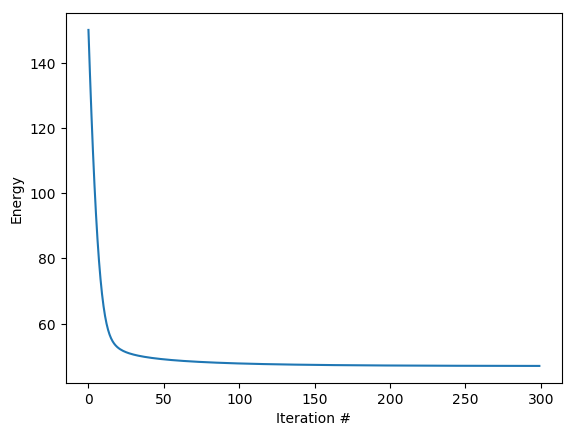Out:
PyObject <matplotlib.text.Text object at 0x00000000277BE898>

Display the result.

In :
imageplot(clamP(fTV))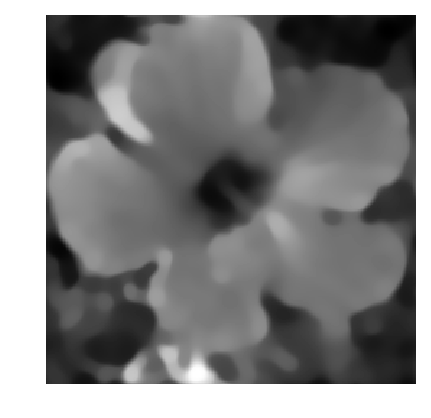### Exercise 4¶

Explore the different values of |lambda| to find the optimal solution. Display the SNR as a function of |lambda|.

In :
include("NtSolutions/inverse_2_deconvolution_variational/exo4.jl")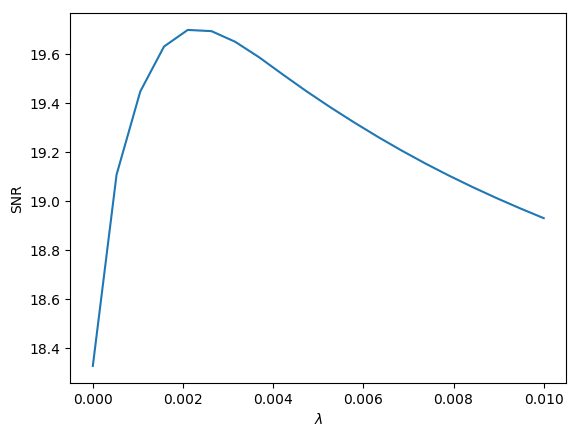Out:
256×256 Array{Float64,2}:
0.172901  0.184695  0.189334  0.192172  …  0.0550672   0.0911487  0.143912
0.146501  0.171049  0.182597  0.188149     0.027206    0.0509994  0.106362
0.123126  0.159742  0.17842   0.18647      0.0122972   0.0212409  0.0756531
0.109446  0.153769  0.177477  0.187861     0.00794715  0.0106811  0.0592337
0.110437  0.157209  0.181602  0.199191     0.00760048  0.0102725  0.0578393
0.12786   0.174116  0.212603  0.224344  …  0.00949967  0.0168529  0.0711875
0.162637  0.229642  0.239075  0.240504     0.0159455   0.03646    0.0963874
0.213519  0.243945  0.24798   0.248833     0.034717    0.05678    0.128953
0.227113  0.24802   0.25198   0.253027     0.0522589   0.0728889  0.154139
0.227243  0.249072  0.253659  0.254802     0.0653509   0.0848381  0.158678
0.218859  0.246976  0.253587  0.25489   …  0.077123    0.0931501  0.150129
0.20009   0.23868   0.251198  0.253374     0.0870894   0.0986414  0.135375
0.171752  0.214282  0.241173  0.249576     0.0941833   0.102005   0.122127
⋮                                       ⋱                         ⋮
0.243645  0.266408  0.272132  0.27442      0.143874    0.153889   0.192127
0.232941  0.263818  0.271575  0.274634  …  0.136259    0.145793   0.178324
0.218862  0.258734  0.269639  0.273994     0.124414    0.133259   0.162822
0.207761  0.252187  0.266322  0.272508     0.112413    0.120254   0.151197
0.205519  0.245682  0.261585  0.269889     0.104098    0.110295   0.148132
0.210889  0.239528  0.255102  0.26534      0.0995502   0.104902   0.155651
0.215267  0.233126  0.246337  0.257285  …  0.0974043   0.105702   0.172506
0.214845  0.226199  0.235345  0.244003     0.0962017   0.118279   0.188907
0.211744  0.219145  0.224139  0.228365     0.0954954   0.13663    0.194512
0.207337  0.212666  0.215214  0.216728     0.0957045   0.145135   0.192853
0.201377  0.205904  0.206979  0.207026     0.0927126   0.140446   0.186037
0.191947  0.197494  0.198267  0.198624  …  0.0797885   0.123041   0.171795 

Display the result.

In :
imageplot(clamP(fBest), string("TV deconvolution, SNR = ", string( round(snr(f0, fBest), 2) ), "dB") )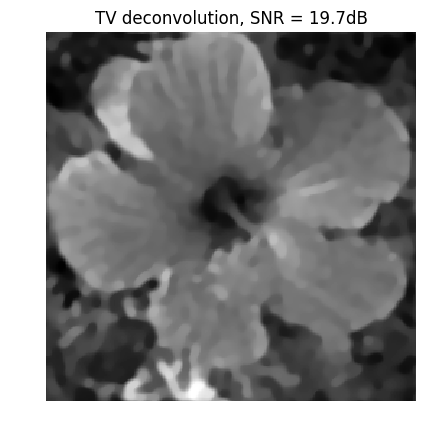Out:
PyObject <matplotlib.text.Text object at 0x000000002A363630>